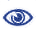# Prague Stringology Conference 2013

Computing Reversed Lempel-Ziv Factorization Online

 Abstract: Kolpakov and Kucherov proposed a variant of the Lempel-Ziv factorization, called the reversed Lempel-Ziv (RLZ) factorization (Theoretical Computer Science, 410(51):5365–5373, 2009). In this paper, we present an on-line algorithm that computes the RLZ factorization of a given string w of length n in O(n log2 n) time using O(n log σ) bits of space, where σ ≤ n is the alphabet size. Also, we introduce a new variant of the RLZ factorization with self-references, and present two on-line algorithms to compute this variant, in O(n log σ) time using O(n log n) bits of space, and in O(n log2 n) time using O(n log σ) bits of space.

 Download paper:PostScript PDF BibTeX reference
 Download presentation: